# 高等微積分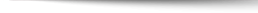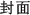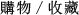## Introduction to Analysis (PNIE) 4/e

+作者：

+年份：
2014 年4 版
+ISBN：
9781292039329
+書號：
MA0463P
+規格：

+頁數：
656
+出版商：
Pearson(Asia)

\$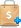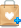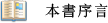Changes to the Exercises
●Computational exercises have been rewritten so that answers are simpler and easier to obtain.
Calculus-style exercises at the beginning of the book have been revised to be more conceptual, emphasizing the same ideas, but at a higher level.
Theoretical exercises of medium difficulty have been added throughout the book.
New True/False questions in the first six chapters confront common misconceptions that students sometimes acquire at this level.
A new section 1.1, Introduction, combines introductory material that was previously scattered over several sections. This section includes two accessible examples about why proof is necessary and why we cannot always trust what we see.
The number of axioms has been reduced from four to three by introducing the Completeness Axiom first, and using it to prove the Well Ordering Principle and the Principle of Mathematical Induction.
The material on countable sets and inverse images of sets has been postponed to Chapter 3, making it possible to begin discussing limits of sequences even earlier than before.
Coverage of Taylor's Formula has been moved from Chapter 7 to Chapter 4 to offer another example of the utility of the Mean Value Theorem.
The Heine-Borel Theorem now has its own section and includes several exercises designed to give students practice in making a local condition on a compact set into a global one.
Section 12.1, Jordan regions, has been organized to simplify the presentation and make it easier to teach.●Flexible presentation, with uniform writing style and notation, covers the material in small sections, allowing instructors to adapt this book to their syllabus.
The practical focus explains assumptions so that students learn the motivation behind the mathematics and are able to construct their own proofs.
Early introduction of the fundamental goals of analysis Refers and examines how a limit operation interacts with algebraic operation.
Optional appendices and enrichment sections enables students to understand the material and allows instructors to tailor their courses.
An alternate chapter on metric spaces allows instructors to cover either chapter independently without mentioning the other.
More than 200 worked examples and 600 exercises encourage students to test comprehension of concepts, while using techniques in other contexts.
Separate coverage of topology and analysis presents purely computational material first, followed by topological material in alternate chapters.
Rigorous presentation of integers provides shorter presentations while focusing on analysis.
Reorganized coverage of series separates series of constants and series of functions into separate chapters.
Consecutive numbering of theorems, definitions and remarks allows students and instructors to find citations easily.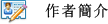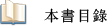1. The Real Number System
2. Sequences in R
3. Continuity on R
4. Differentiability on R
5 Integrability on R
6. Infinite Series of Real Numbers
7. Infinite Series of Functions
8. Euclidean Spaces
9. Convergence in Rn
10. Metric Spaces
11. Differentiability on Rn
12. Integration on Rn
13. Fundamental Theorems of Vector Calculus
14. Fourier Series
Answers and Hints to Selected Exercises